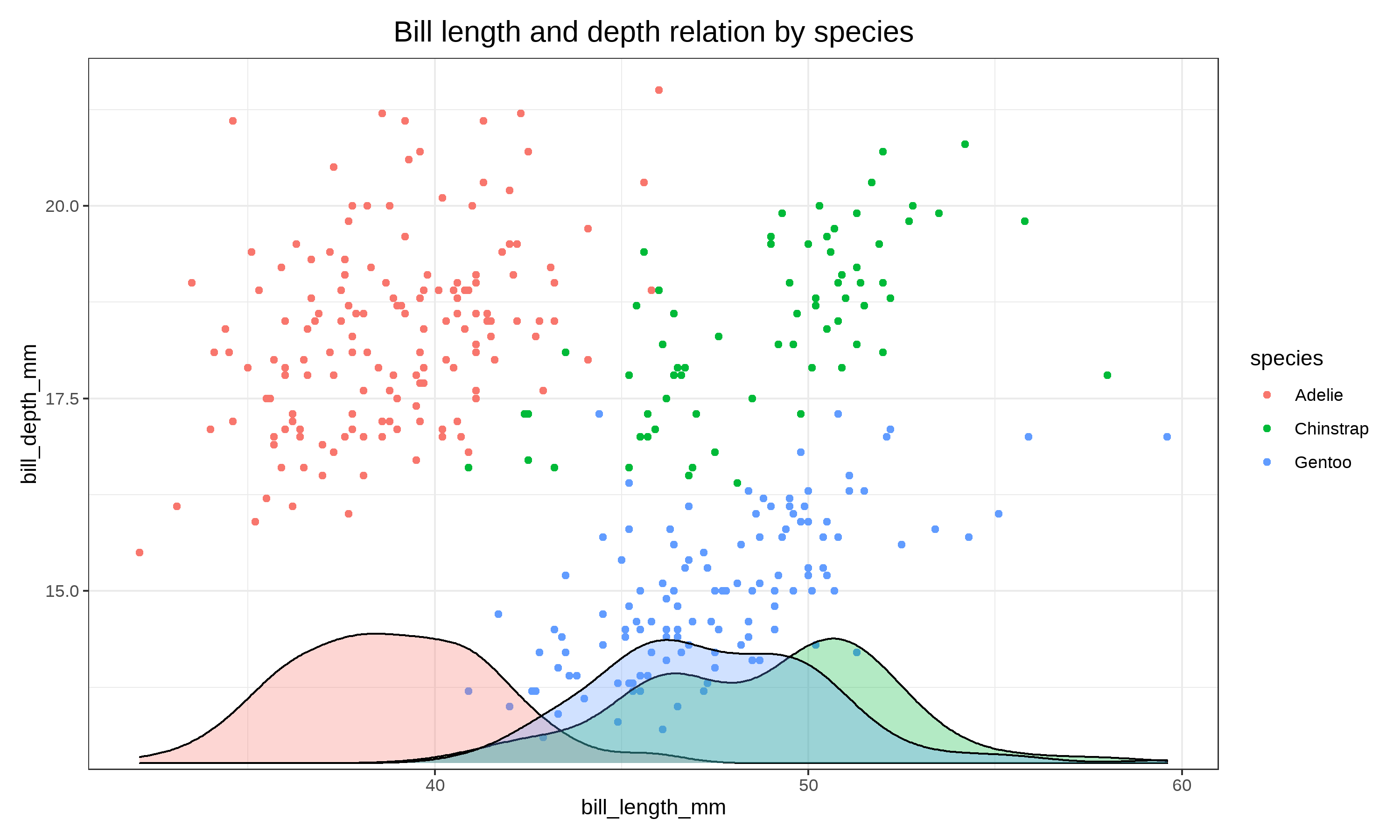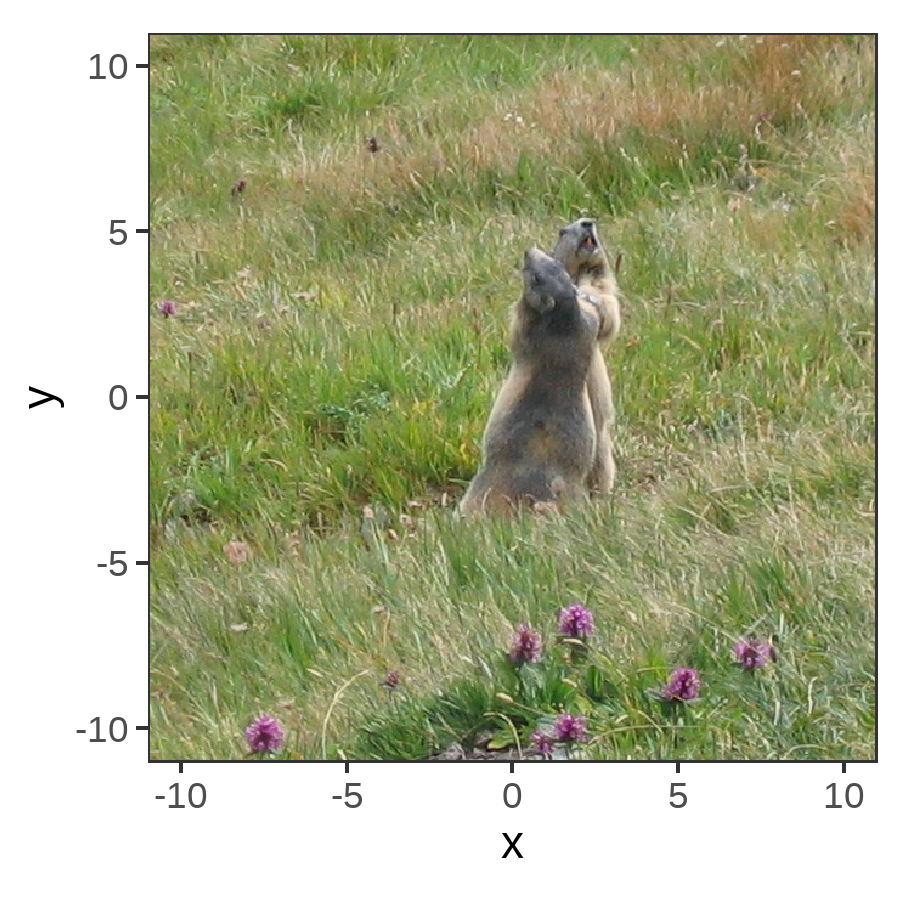# Relative coordinates or NPC in ggplot2

Normalized Parent Coordinates

In ggplot2 we use the coordinate system to place the element in the plot. But sometime we need to organise multiple plots or place images, and we place the element relative to a parent element, and we use a different coordinate system : “Normalized Parent Coordinates” or NPC.

So what is NPC ? NPC means that the parent coordinates is normalized from 0 to 1. So the plot has the origin (0, 0) at bottom left corner, and the top right corner at the coordinate (1, 1), the maximum size is 1 of both axis.

## Positionning element with patchwork

We can look at an example by combining two plots with the package “patchwork”.

``````library(ggplot2)
library(dplyr)
library(palmerpenguins)
library(patchwork)

theme_set(theme_bw())

density <- penguins %>%
ggplot(aes(bill_length_mm, fill = species)) +
geom_density(alpha = 0.3) +
theme_void() +
guides(fill = FALSE)

plt <- penguins %>%
ggplot(aes(bill_length_mm, bill_depth_mm, color = species)) +
geom_point()+
labs(title = "Bill length and depth relation by species") +
theme(plot.title = element_text(size = 15, hjust = 0.5))

final <- plt + inset_element(density, left = 0, bottom = 0, right = 1, top = 0.2, align_to = "panel")

ggsave(here::here("render", "plot_patchwork.png"),
plot = final, width = 10, height = 6, units = "in", dpi = 300)``````We tell patchwork that the reference is the panel, so the size of the panel (parent) is normalized from 0 to 1. We want to display the density plot aligned to the full panel, so from left = 0 to right = 1, and a height only 1/5, so bottom = 0 to top = 0.2

You can select what the coordinates should be relative to with align_to. Here I used panel, because I wanted my density plot to be aligned with the scatterplot. But you can use “plot” (panel + axis) or “full” (full image).

## A mixed example with ggimage

Sometimes it can be confusing to work with coordinate system, because you can mix the two coordinates system to place and size correctly an element. Let’s have a look by positioning an image with ggimage.

Let’s place an image in the center of a plot, with a size of 1 :

``````library(ggimage)

img <- here::here("input", "marmottes.png")

ggplot()+
geom_image(aes(x = 0, y = 0, image = img), size = 1) +
scale_x_continuous(limits = c(-10,10)) +
scale_y_continuous(limits = c(-10,10))``````The image is positioned at (0,0) on the coordinate system of the plot, as we could expect, but it takes all the size on each axis, and the picture takes the whole panel.

Here the size is normalized from 0 to 1 of the size of the panel.

If we indicate a size of 0.5, the image is half the size on both axis, so 25% of the plot area :

``````ggplot()+
geom_image(aes(x = 0, y = 0, image = img), size = 0.5) +
scale_x_continuous(limits = c(-10,10)) +
scale_y_continuous(limits = c(-10,10))``````If the axis have a different size, the image is stretched.

``````ggplot()+
geom_image(aes(x = 0, y = 0, image = img), size = 0.5) +
scale_x_continuous(limits = c(-10,10)) +
scale_y_continuous(limits = c(-20,20)) +
coord_fixed(ratio = 1)``````So we can set the reference for the size and keep an aspect ratio, we can tell geom_image that the reference is the “width” using the `by` parameter, and fixing an aspect ratio, `asp = 0.5`.

``````ggplot()+
geom_image(aes(x = 0, y = 0, image = img), by = "width", size = 0.5, asp = 0.5) +
scale_x_continuous(limits = c(-10,10)) +
scale_y_continuous(limits = c(-20,20)) +
coord_fixed(ratio = 1)``````# wrap-up

Some elements use the normalized parent coordinates system for positioning and size with bottom, top, left, right dimensions.

Be aware that in the example with geom_image, the positioning is still with the coordinate system of the plot, and the size is in npc whereas With inset_element in patchwork, the positioning with left, top, bottom, right is to define the area in npc.

It is therefore important to be aware of the existence of different coordinate systems when using a new package, to quickly become comfortable with positioning and scale.##### Christophe Nicault
###### Information System Strategy Digital Transformation Data Science

I work on information system strategy, IT projects, and data science.